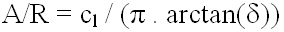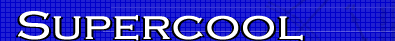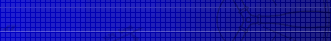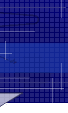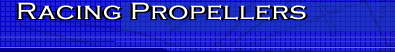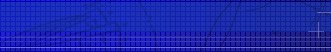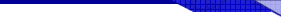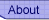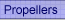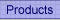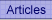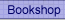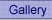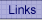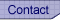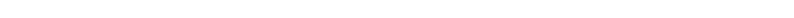﻿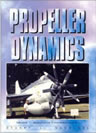Propeller Dynamics Essential reading for model aircraft contest fliers. This is the only book on the market explaining propeller theory in non-mathematical terms. A rattling good read, I know, I wrote it. Read more...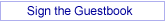## Analytical Design of Propellers - part 3 Induced angle of attack

By Joe Supercool

In the first and second articles of this series, I have introduced the notion that one can have immediate success in the design of propellers if one already knows their design parameters and performance. This method is like having 100% hindsight: it is much better than thrashing around with complex equations full of approximations and uncertainties. You know, garbage in, garbage out.

Essentially, there is just one unknown in our retrograde world: that is the angle of attack of the airfoil to the undeflected free-stream. We need both this angle of attack and the associated lift coefficient to complete our “design” process.

For a brief historical diversion, it’s worth noting that this is an “old” problem, being sorted out prior to 1930. I can do no better than quote directly from “Aircraft Propeller Design” by Fred E. Weick, B.S., an aeronautical engineer from the NACA Langley Memorial Laboratory, Virginia, USA, 1930.

“ In the application of the airfoil theory to propeller blade-element analysis, the two-dimensional or profile characteristics are used for the airfoil elements, and the interference flow is calculated from the momentum generated, after the manner of Froude and Rankine. Thus, since only the profile lift and profile drag are used, the questionable aspect ratio effect is eliminated. Also, since the interference flow is determined by the number of blades, their spacing, and the forces on each, the blade interference is accounted for”.

Now that is a mouthful. Ignoring the Americanised grammar, that says it all.
Says what?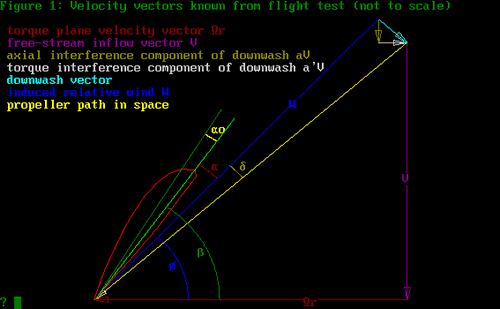Figure 1

Refer to Figure 1. There are 3 angles shown here: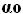,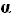and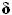. The first,, is the airfoil zero-lift angle, measured to the chord-line. To obtain, I use the method of R.T. Jones, “Modern Subsonic Aerodynamics”, 1988: viz,= arctan(m/(1-p)), where m is the camber and p the camber-line high point. This method agrees very well with the more complex procedure given in Abbott and Von Doenhoff.

The second angle,, is the angle of attack for an airfoil of infinite aspect ratio. In the presentation of data for airfoils, with angle of attack plotted against lift coefficient (the lift-slope), this is the value that is presented. Just what it is in reality, we may debate!

The third angle,, is the reduction in inflow angle due to the downwash. If that makes no sense to you, just look on the diagram. It still won’t make sense, but at least you know where it is.

Now at this stage, we are following the vector diagram given by Larrabee and others quite well. But what it all means is by no means clear. Recall, we are trying to find the angle of attack to use with our expression from Part 2, viz: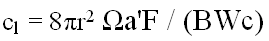The terms a' and W in the above equation are dependent on the angle of attack, if we only knew what angle we are talking about.

Now as it happens, Larrabee says this: “The necessary geometric angle with respect to the undisturbed air is obtained by adding the induced angle of attack to the theoretical angle of attack required for a wing of infinite span in two-dimensional (Joukowsky) flow." (Scientific American, July 1980, “The Screw Propeller”)

OK. That settles the matter. Now it’s all clear. Right? From our diagram, just addand. But to do what? Weick tells us to get Cl from the two-dimensional data, namely usingonly. Maybe we need a diagram. David Rehm’s article “Understanding Airfoil Data”, the Experimenter via Pacific Flyer, March 2004, is a good start for further reading. Blame him for the following.

Rehm states: “When the wing is at zero-lift, this is the one and only point where a 2-D wing (ed: infinite aspect ratio) and a 3-D (ed: finite aspect ratio) wing will behave the same…they will both produce zero lift. Start pitching up with a 3-D wing and the lift slope will rotate downward (ed: see my Figure 2). The important factor is that they rotate about the point where they cross the horizontal line at zero lift coefficient”

The above statement by Rehm allowed me to draw Figure 2. All I had to do was take the point at ( -, 0) and rotate the lift slope clockwise to form the lift slope for an airfoil of finite aspect ratio. This is a legitimate lift-slope, which we can construct for our guessed values ofand.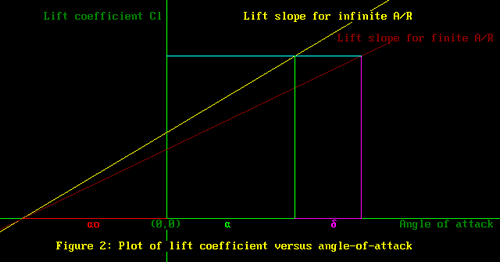Figure 2

We required the guessed values to give us our design lift coefficient. Now it can be seen that this value of lift coefficient is achieved when Cl for angle-of-attackfor infinite as aspect ratio lift slope is the same for the finite aspect ratio case when the angle of attack is+.

Therefore, it makes no difference which lift slope we use. However, the only data we have is for the lift slope data for infinite aspect ratio, so I guess that means we have no choice.

There is an interesting corollary to all this. Some folks like to say, look at that long thin prop, it must have a high aspect ratio. Good point.

Using the definition that converts low aspect ratio (A/R) data to infinite aspect ratio, we can show that for a given blade element,